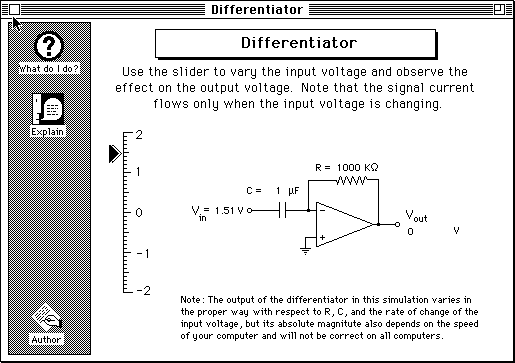Differentiator

The purpose of this circuit is to generate an output voltage Vout that is proportional to the rate at which the input voltage Vin is changing. That is, if the input voltage is changing slowly, the output voltage is low; if the input voltage is changing quickly, the output voltage is higher. Increasing input voltage results in a negative output voltage; decreasing input voltage results in a positive output voltage. If the input voltage is constant, the output is zero. From a mathematical point of view, the output voltage is the (negative of the) derivative of the input signal with respect to time (-dVin/dt), hence the name of the circuit.

One of the practical applications of this circuit is to monitor the constancy of signals and to warn when there are changes (as in motion detectors in security systems). Another application is to determine the location of the maxima of the peaks in recorded spectra or chromatograms (when the input voltage goes through a maximum, the output voltage swings from negative to positive). Two of these circuits can be used in series to determine the location of the inflection point in a sigmoidal curve (e.g. to automatically locate the end-point in a titration curve).

The slider controls the input voltage. Note that the signal current (shown by arrows) flows only when the input voltage is changing.

From the definition of capacitance, the current through the capacitor is given by C(dVin/dt). (The inverting input of the op amp is virtual ground). The capacitor current flows only through the feedback resistor R (for the usual reasons). The arrows show the direction of current flow. The current flowing through the feedback resistor generates an output voltage equal to -RC(dVin/dt).

Note: The output of the differentiator in this simulation varies in the proper way with respect to R, C, and the rate of change of the input voltage, but its absolute magnitute also depends on the speed of your computer and will not be correct on all computers.